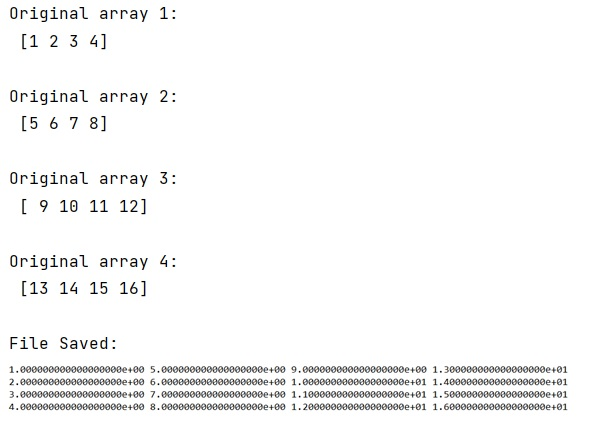# How to save arrays as columns with numpy.savetxt()?

Learn, how to save arrays as columns with numpy.savetxt() in Python?
Submitted by Pranit Sharma, on February 16, 2023

NumPy is an abbreviated form of Numerical Python. It is used for different types of scientific operations in python. Numpy is a vast library in python which is used for almost every kind of scientific or mathematical operation. It is itself an array which is a collection of various methods and functions for processing the arrays.

## Saving arrays as columns with numpy.savetxt()

Suppose that we are given 4 numpy 1D arrays and we need to save these into a text file using savetxt file. One of the possible problems which we might face is that all the arrays are saved row-wise. We need to save these arrays as columns.

For this purpose, we will use np.c_. This method translates slice objects to concatenation along the second axis.

This is short-hand for np.r_['-1,2,0', index expression], which is useful because of its common occurrence. In particular, arrays will be stacked along their last axis after being upgraded to at least 2-D with 1's post-pended to the shape (column vectors made out of 1-D arrays).

Let us understand with the help of an example,

## Python code to save arrays as columns with numpy.savetxt()

```# Import numpy
import numpy as np

# Creating numpy arrays
a = np.array([1, 2, 3, 4])
b=np.array([5,6,7,8])
c=np.array([9,10,11,12])
d=np.array([13,14,15,16])

# Display original arrays
print("Original array 1:\n",a,"\n")
print("Original array 2:\n",b,"\n")
print("Original array 3:\n",c,"\n")
print("Original array 4:\n",d,"\n")

# Saving all the arrays as column in txt file
np.savetxt('myfile.txt', np.c_[a,b,c,d])

# Display a msg
print("File Saved:\n")
```

Output: